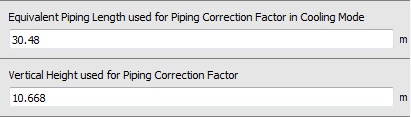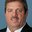Question-and-Answer Resource for the Building Energy Modeling Community
Get s tarted with the Help page

# Determining "Equivalent Piping Length used for Piping Correction Factor" for VRFWhen entering VRF inputs in an OpenStudio model, there are equivalent length inputs used to estimate piping losses.Does the "Equivalent Piping Length in Cooling Mode" represent the length of the farthest terminal unit from the outdoor unit and should this typically be equal to the "Equivalent Piping Length use for Piping Correction Factor in Heating Mode"?

The Engineering Reference documentation gives a definition of the equivalent length in the heating mode section; I didn't see it in the cooling mode section but I'm assuming it's meant to represent the same thing (i.e., piping length to the farthest terminal unit).

edit retag close merge delete

Sort by » oldest newest most votedYou are correct in that this input is meant to represent the farthest terminal unit and therefore the expected piping losses.

more